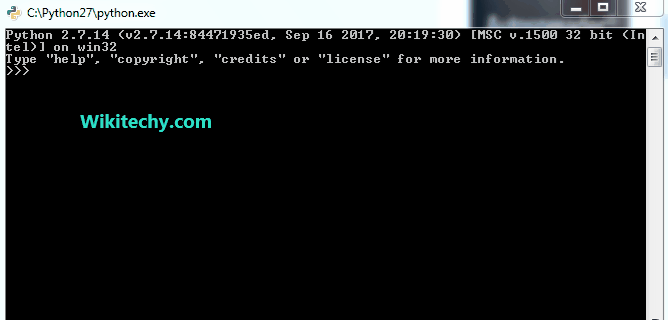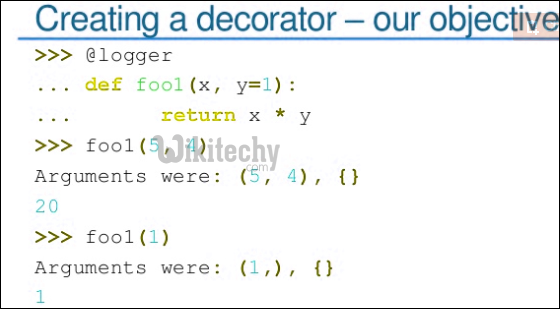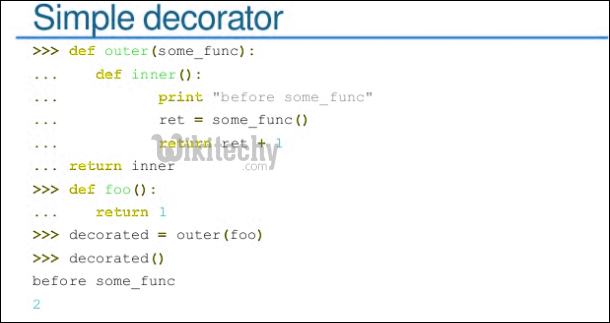# python tutorial - Python Decorators | Function Decorators in Python | Introduction - learn python - python programming

## Decorators

• "Decoration is a way to specify management code for functions and classes." ... "A decorator itself is a callable that returns a callable."
• A function object is a callable. So, the previous statement can be translated into:
• A decorator is a function that takes a function object as its argument, and returns a function object, and in the process, makes necessary modifications to the input function, possibly enhancing it.
• Decorator wraps a function without modifying the function itself. The result of the wrapping?
• Adds functionality of the function.
• Modifies the behavior of the function.

## Function Decorators

• Let's start with a function in Python. To understand decorators, we need to know the full scope of capabilities of Python functions.
• Everything in Python is an object. Function is not an exception.
• As we can see, f() is an object, and it's not different from classes (MyClass) or variables (a).
• We can assign a function to a variable, so the following lines of code is legally perfect.
• Functions can be passed around in the same way other types of object such as strings, integers, lists, etc.
• Another face of a function in Python is it can accept a function as an argument and return a new function object as shown below.
• The myFunction is indeed a decorator because by definition a decorator is a function that takes a function object as its argument, and returns a function object.
• If we elaborate a little bit more on the function we just defined:

## How we invoke our decorator ?

• Let's look at the example below. We put a simple_function into the decorator (myFunction) as an argument, and get a enhanced_function as a return value from the decorator.
• In many cases, we use the same name for the returned function objects as the name of the input function. So, practically, the code should look like this:
• If we apply the decorator syntax to the code above:
• Note that the first line @myFunctionas is not a decorator but rather a decorator line or an annotation line, etc.
• The @ indicates the application of the decorator. A decorator is the function itself which takes a function, and returns a new function. In our case, it is myFunction.
• When the compiler passes over this code, simple_function() is compiled and the resulting function object is passed to the myFunction code, which does something to produce a function-like object that is then substituted for the original simple_function().
• Also, note that in the line:
• The decorator(myFunction) is rebinding function name to decorator result.
• So, when the simple_function is later called, it's actually calling the object returned by the decorator.
• We've seen the rebinding when we define a static method:
• The equivalent code using decorator looks like this:
• Another example: suppose we have two functions defined this way:
• Then, the wrapper can be used for rebinding foo() like this:
• So, it's a decorator:
• With a decorator defined as below:
• it automatically maps the following:
• into the equivalent form as shown below:
• The decorator is a callable object that returns a callable object with the same number of argument as f.
• So, decoration maps the following line:
• into

## Background

Following are important facts about functions in Python that are useful to understand decorator functions.

1. In Python, we can define a function inside another function.
2. In Python, a function can be passed as parameter to another function (a function can also return another function).Learn Python - Python tutorial - python functions - Python examples - Python programs

python - Sample - python code :

Output:

`Welcome to Wikitechy`

Function DecoratorA decorator is a function that takes a function as its only parameter and returns a function. This is helpful to “wrap” functionality with the same code over and over again. For example, above code can be re-written as following.We use @func_name to specify a decorator to be applied on another function.python - Sample - python code :

Output:

`Welcome to Wikitechy`Decorators can also be useful to attach data (or add attribute) to functions.

python - Sample - python code :

Output:

```5
3```

‘add()’ returns sum of x and y passed as arguments but it is wrapped by a decorator function, calling add(2, 3) would simply give sum of two numbers but when we call add.data then ‘add’ function is passed into then decorator function ‘attach_data’ as argument and this function returns ‘add’ function with an attribute ‘data’ that is set to 3 and hence prints it.

Python decorators are a powerful tool to remove redundancy.

Wikitechy tutorial site provides you all the learn python , learn to program in python , python coding class , learn python programming language online , learn python coding , learn how to code python , free python course online , python training online free , python book , python developer , python online course free